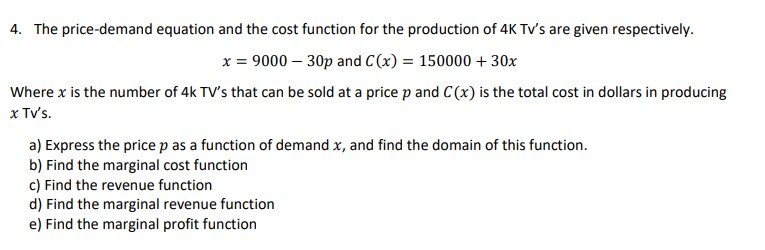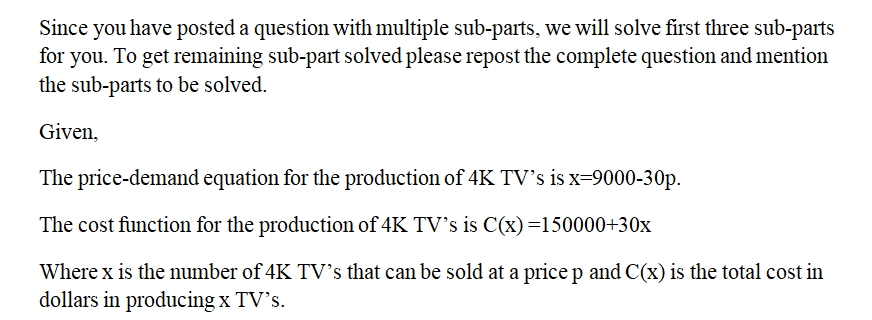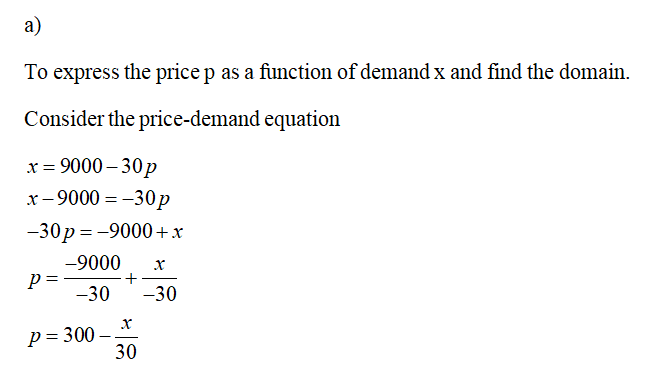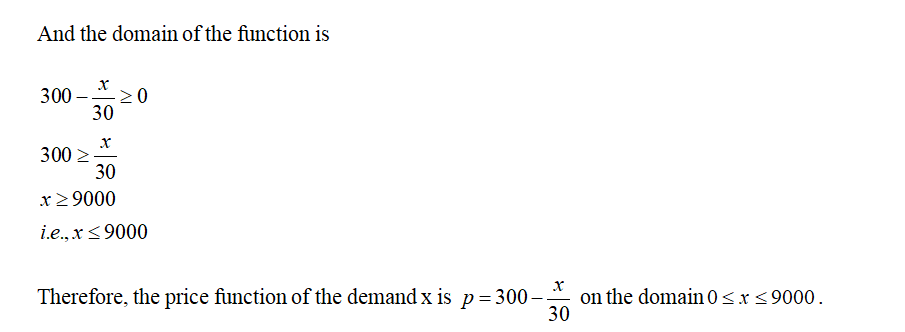# 4. The price-demand equation and the cost function for the production of 4K Tv's are given respectively.x = 9000 – 30p and C(x) = 150000 + 30xWhere x is the number of 4k TV's that can be sold at a price p and C(x) is the total cost in dollars in producingx TV's.a) Express the price p as a function of demand x, and find the domain of this function.b) Find the marginal cost functionc) Find the revenue functiond) Find the marginal revenue functione) Find the marginal profit function

Question
17 viewshelp_outlineImage Transcriptionclose4. The price-demand equation and the cost function for the production of 4K Tv's are given respectively. x = 9000 – 30p and C(x) = 150000 + 30x Where x is the number of 4k TV's that can be sold at a price p and C(x) is the total cost in dollars in producing x TV's. a) Express the price p as a function of demand x, and find the domain of this function. b) Find the marginal cost function c) Find the revenue function d) Find the marginal revenue function e) Find the marginal profit function fullscreen
check_circle

Step 1Step 2Step 3...

### Want to see the full answer?

See Solution

#### Want to see this answer and more?

Solutions are written by subject experts who are available 24/7. Questions are typically answered within 1 hour.*

See Solution
*Response times may vary by subject and question.
Tagged in

### Calculus